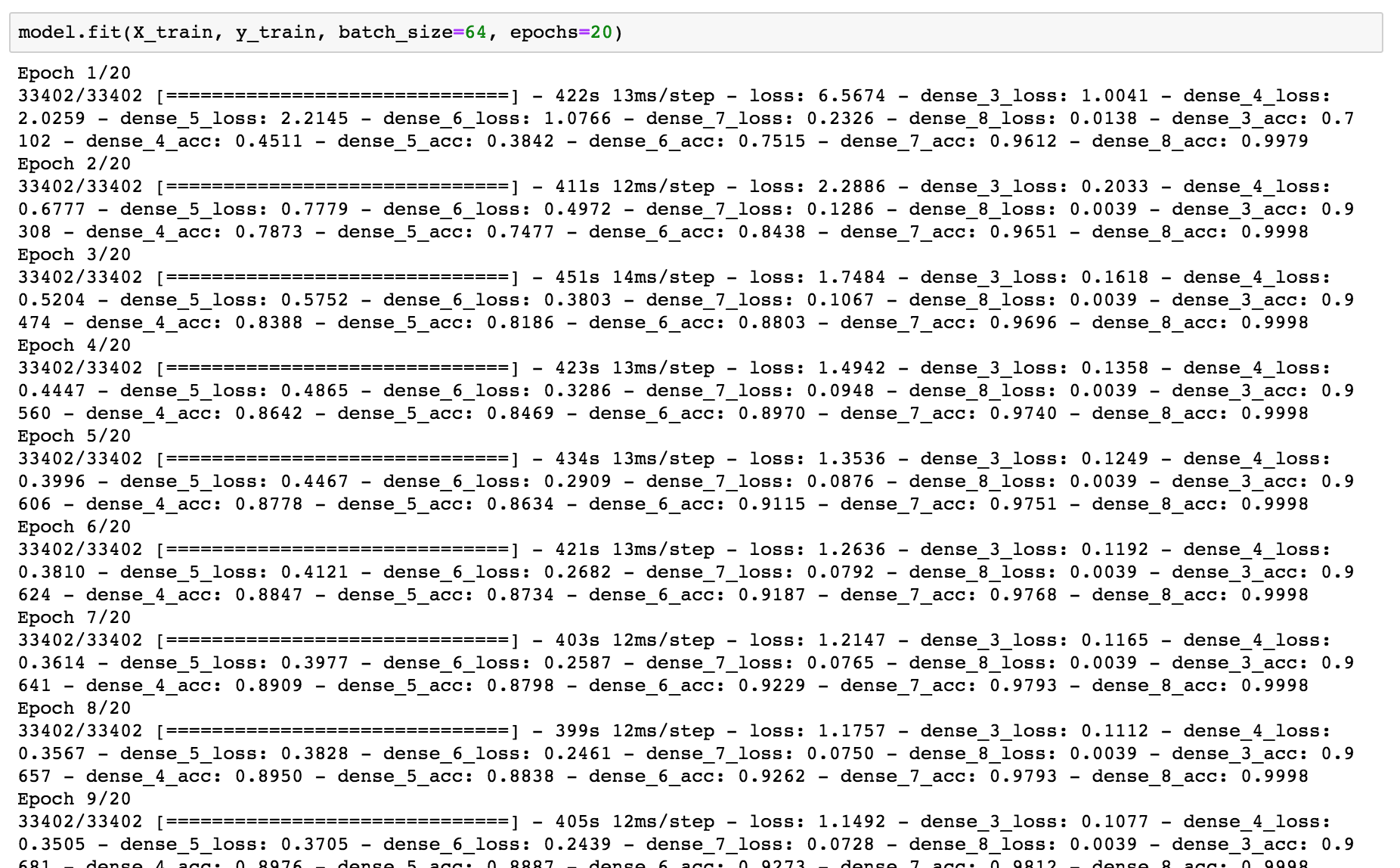## 任务要求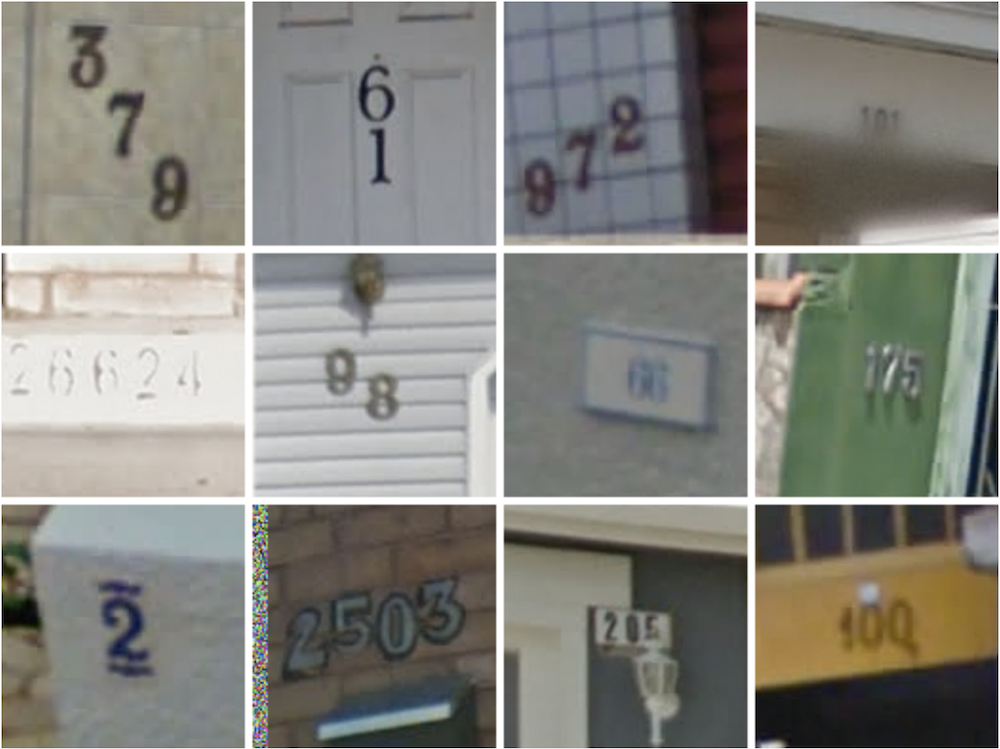## 过去的做法

Traditional approaches to solve this problem typically separate out the localization, segmentation, and recognition steps.（过去的做法要经历三步：定位，分割，然后识别）

## 作者的做法

Propose a unified approach that integrates these three steps via the use of a deep convolutional neural network that operates directly on the image pixels.（作者把这三个步骤通过一个深度的卷积网络就能完成）

## 作者的贡献

• (a) A unified model to localize, segment, and recognize multi-digit numbers from street level photographs
• (b) A new kind of output layer, providing a conditional probabilistic model of sequences
• (c) Empirical results that show this model performing best with a deep architecture
• (d) Reaching human level performance at specific operating thresholds.

## 实现方法

• S：输出序列，也就是训练数据的label。
• X：输入的图片。

X其实就是输入的图片，这里看一下S，S是：图片的数字序列$$S_1,…,S_N$$ + 数字序列的长度$$L$$的一个集合。比如上面的”379”是图片的数字序列，序列的长度len(“379”)为3。那么S就是”3”+”379”，也就是”3379”。

## 模型结构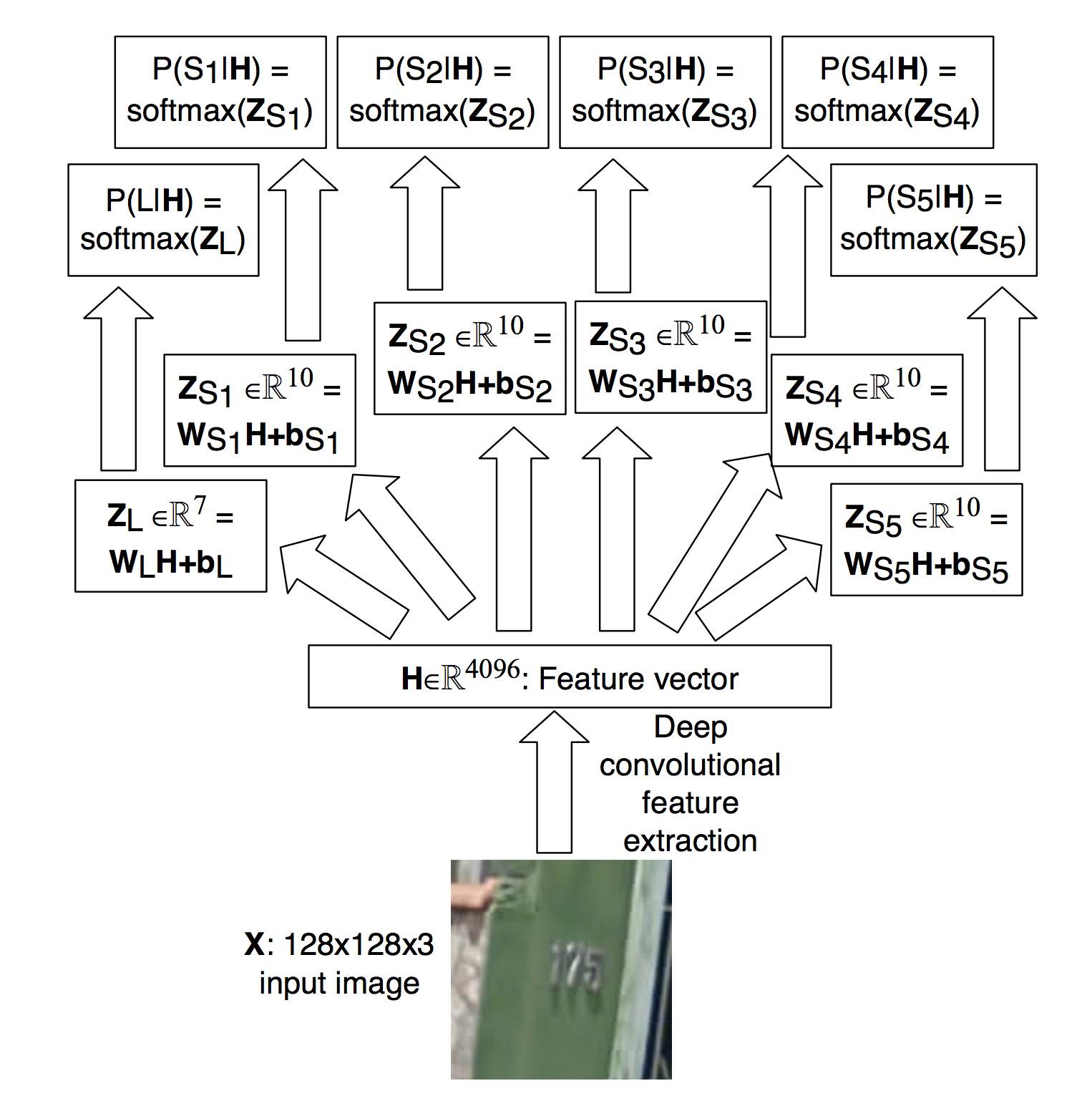• 输入图片$$X$$是一个128x128x3的图片。
• 然后经过一系列的CNN层进行特征提取，变成了一个含有4096个特征的向量。
• 之后根据这4096个特征，分别让$$L$$、$$S_1$$、$$S_2$$、$$S_3$$、$$S_4$$、$$S_5$$分别经过一个Softmax层$$P(S_i|H)=softmax(W_{S_i}H+b_{S_i})$$。
• 对于每个变量，$$s_i = \arg\max_{S_i}logP(S_i | H).$$

## Keras的实现

### 环境依赖

• python 3.x
• TensorFlow 1.11
• Keras 2.x
• Pillow
• h5py

### 构建数据集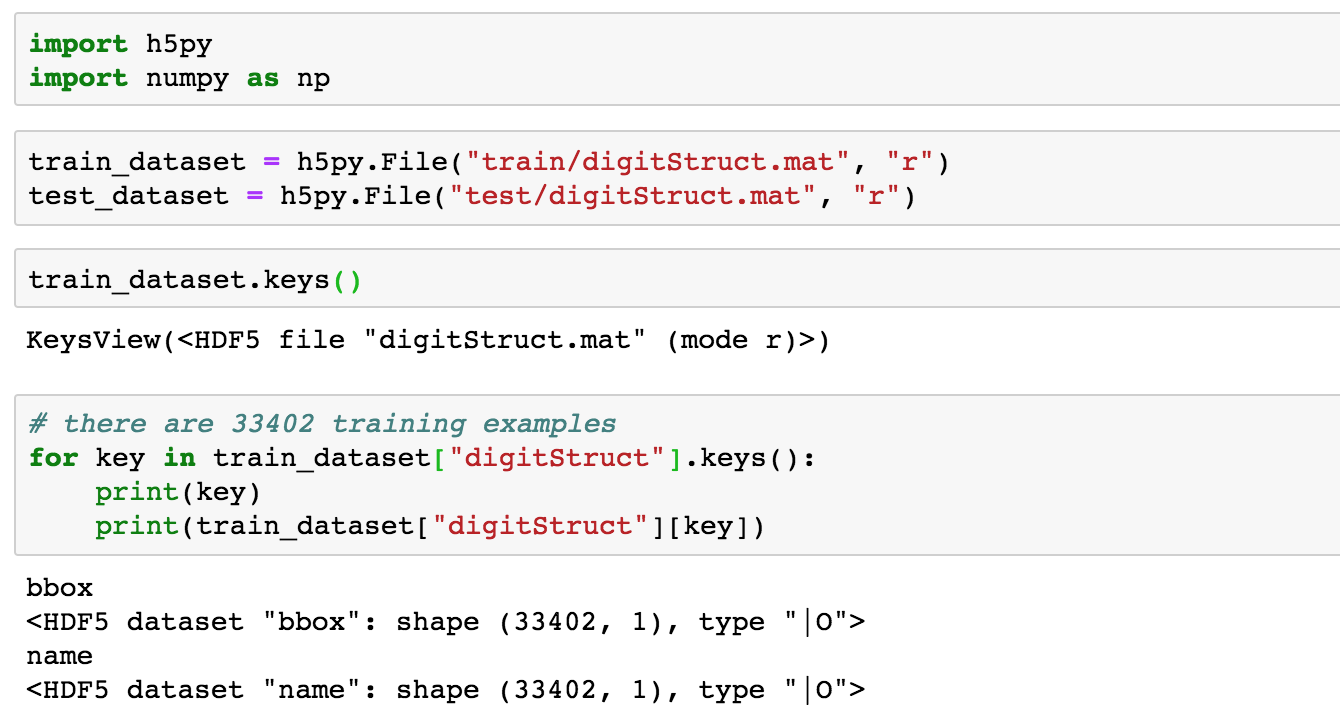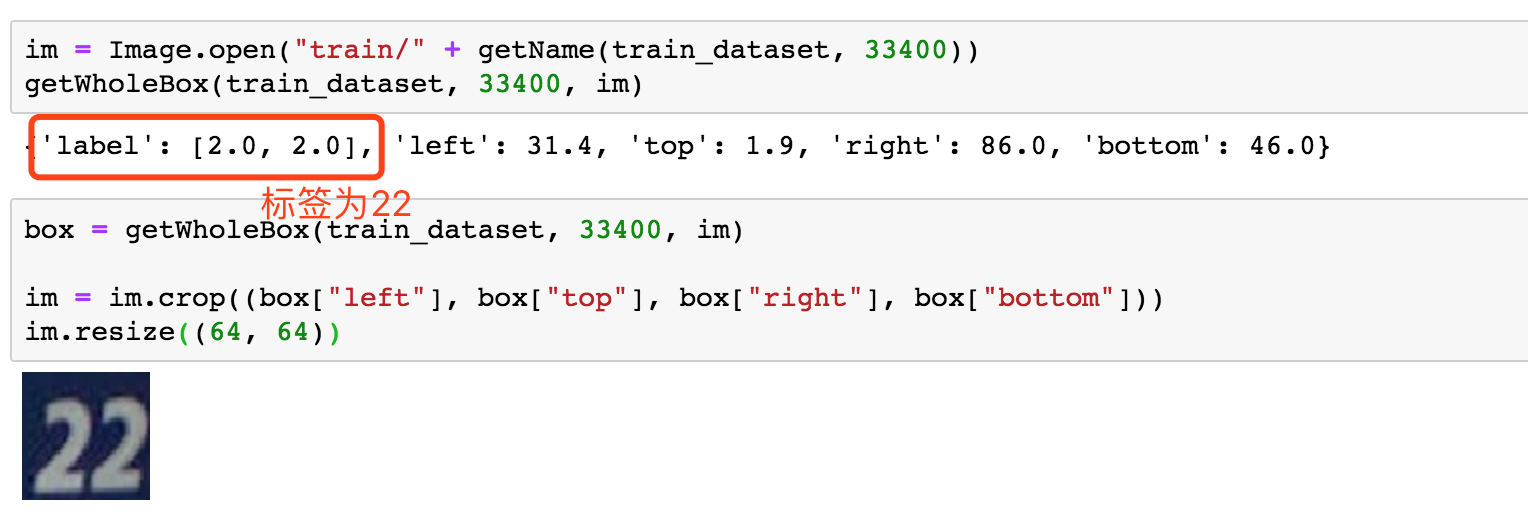### 网络模型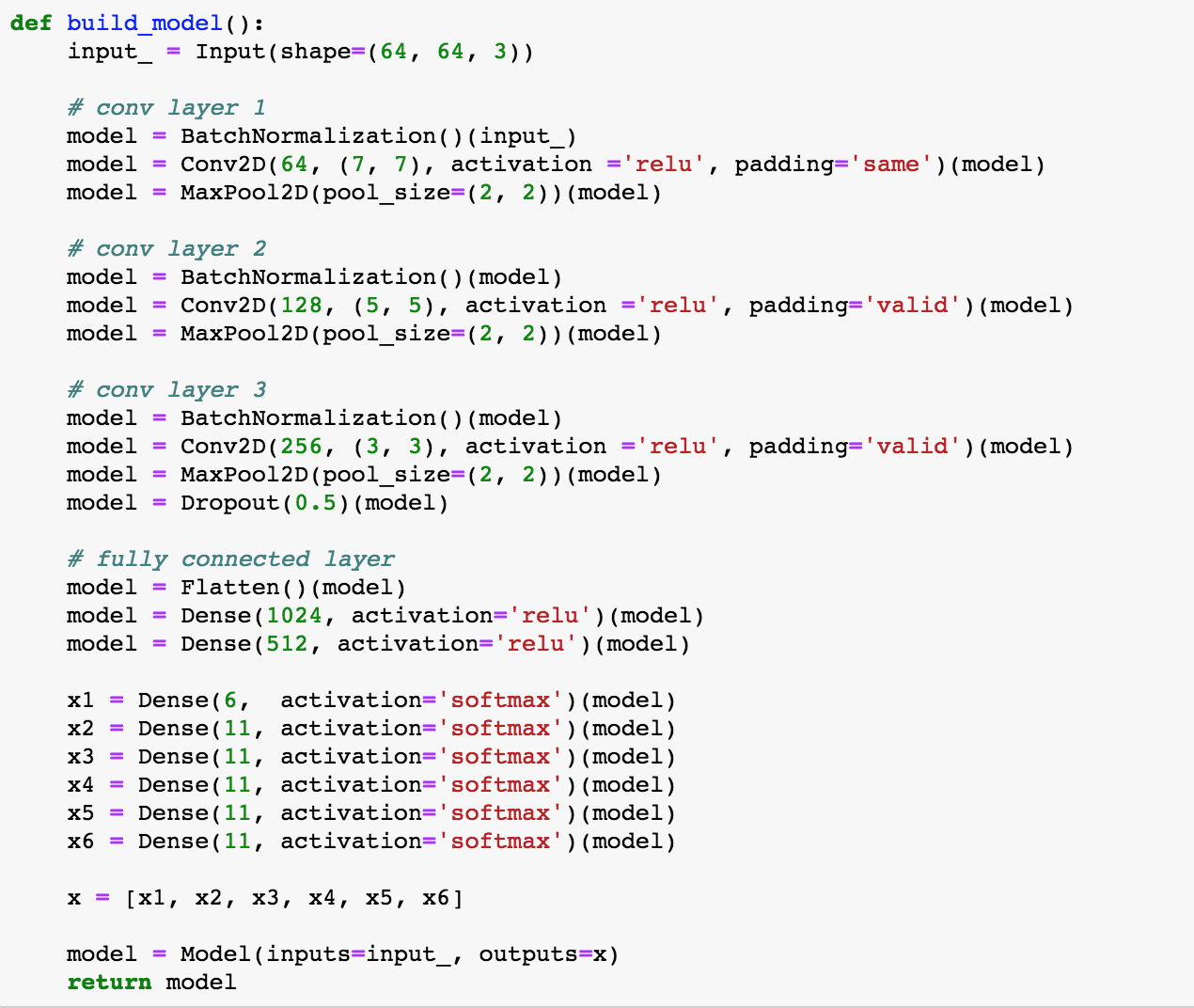### 训练与测试